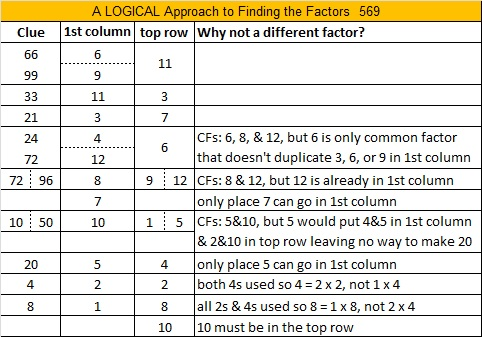# 569 and Level 5

When we divide the last two digits of prime number 569 by 4, we get a remainder of one. That means that 569 is the sum of two square numbers, specifically, 20² + 13² = 569.

From those two square numbers we will obtain 569 as the hypotenuse of the primitive Pythagorean triple 231-520-569:

• 20² – 13² = 231
• 2 x 13 x 20 = 520
• 20² + 13² = 569Print the puzzles or type the solution on this excel file: 12 Factors 2015-07-27

—————————————————————————————————

• 569 is a prime number.
• Prime factorization: 569 is prime.
• The exponent of prime number 569 is 1. Adding 1 to that exponent we get (1 + 1) = 2. Therefore 569 has exactly 2 factors.
• Factors of 569: 1, 569
• Factor pairs: 569 = 1 x 569
• 569 has no square factors that allow its square root to be simplified. √569 ≈ 23.85372How do we know that 569 is a prime number? If 569 were not a prime number, then it would be divisible by at least one prime number less than or equal to √569 ≈ 23.8. Since 569 cannot be divided evenly by 2, 3, 5, 7, 11, 13, 17, 19, or 23, we know that 569 is a prime number.This site uses Akismet to reduce spam. Learn how your comment data is processed.